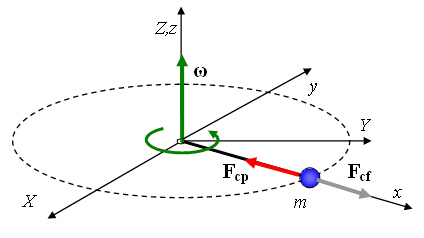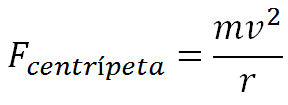# Centripetal Force: Definition, Origin and Everyday ExamplesCentripetal force is a physical concept that plays a crucial role in kinematics and, in particular, circular motion.

Despite its importance in understanding everyday and natural phenomena, it is often misunderstood or confused with centrifugal force.

In this article, we will explain what centripetal force is, its applications in everyday life and how it relates to circular motion.

## What Is Centripetal Force?Centripetal force is a force that acts on an object moving along a circular path. Its direction always points towards the center of the circle in which the object moves. This force is necessary to keep the object moving in a circular path instead of moving in a straight line.

It is often confused with "centrifugal force", but it is important to understand that centrifugal force is rather an apparent illusion caused by the inertia of objects in circular motion.

## The Origin of Centripetal Force

Centripetal force has its roots in the fundamental laws of physics, particularly Newton's second law. According to this law, the acceleration of an object is directly proportional to the force applied and is inversely proportional to its mass.

In the context of circular motion, this translates into a need for a net force toward the center of the circle to vary the rectilinear path.

To better understand this concept, let's consider the classic example of a spinning rope with a mass tied to the end. As the object at the end of the string rotates, it experiences a centripetal force that holds it in its orbit.

This force originates from the tension in the string and acts as a net force directed toward the center of the circle of rotation.

## Centripetal Force Formula

The centripetal force is calculated using the following equation:Where:

• Fc​ is the centripetal force.

• m is the mass of the moving object.

• v is the tangential velocity of the object.

• r is the radius of the circular path.

This equation shows that the centripetal force is directly proportional to the mass of the object and the square of its tangential velocity. Furthermore, the centripetal force is inversely proportional to the radius of the circular path.

In other words, at a higher tangential speed or a smaller radius, a greater centripetal force will be required to keep the object in its circular orbit.

## 6 Examples of Centripetal Force

Centripetal force is a fundamental part of many aspects of everyday life and modern technology.

Here are some notable examples:

### 1. Vehicles on Curves

When a car travels through a curve in the road, it experiences a centripetal force that keeps it on track. The centripetal force needed depends on the speed of the car, the radius of the curve, and the mass of the vehicle.

### 2. Roller Coasters

When a roller coaster car travels through loops and turns, it experiences centripetal forces that keep it moving along the track.

The fun and excitement of a roller coaster is due, in part, to these controlled centripetal forces.

### 3. Cycling and MotorcyclingBicyclists and motorcyclists rely on centripetal force to stay in position when cornering. Leaning toward the inside of the curve allows centripetal force to balance the rider's inertia, keeping them on the path without falling.

### 4. Centrifuges

Centrifuges are devices used in laboratories and industry to separate components of a mixture based on their density.

Centripetal force is applied to the rotating sample, causing the heavier particles to move to the bottom and the lighter particles to move upward, thus allowing separation.

### 5. Satellites and Spacecraft

Satellites and spacecraft in orbit around the Earth or other celestial bodies depend on centripetal force to stay in their orbits. This force counteracts gravitational attraction and allows space objects to remain in constant orbital motion and is an important component in the study of astronomy.

### 6. Wind Turbines

Wind energy is a type of energy that converts wind energy into electricity. This function is performed by wind turbines whose blades must be designed taking into account the centripetal force that they will have to withstand when they are rotating at full capacity.